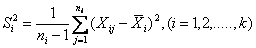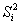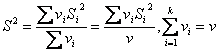# Statistics Assignment Help With Bartlett's Test For Homogeneity

## 11.10 Bartlett's Test For Homogeneity Of Several Independent Estimates Of The Same Population Variance:

LetBe the unbiased estimate of the population variance, obtained from the ith sample Xij,(j=1, 2, …….ni)and based on vi = (ni – 1) degrees if freedom, all the k samples being independent.

Under the null hypothesis that the samples come from the same population with variance σ2, that is the independent estimates, (i =1,2,….k) of σ2are homogeneous, Bartlet proved that the statisticWhereFollows chi-square distribution with (k-1) degrees of freedom.